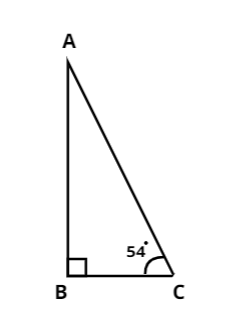Courses
Courses for Kids
Free study material
Offline Centres
MoreLast updated date: 09th Dec 2023
Total views: 382.8k
Views today: 8.82k

# One of the acute angles of a right triangle is 540, find the other acute angle.Verified
382.8k+ views
Hint: In this question we need to find the third angle of a right angle triangle, so to solve this question we will use the angle sum property of triangles. This property will help us simplify the question and eventually help us reach the answer.

We have been given a right triangle and it's one of the acute angles is $54^o$.
So, let us assume a right angled triangle ABC, which is right angled at B and $\angle C = {54^0}$ like in the given figure.So, from the above figure we need to find $\angle A$.
Now, using the Angle Sum property of Triangle we get,
$\angle A + \angle B + \angle C = {180^0}$ ……………. Equation (1)
As the given triangle is right angled triangle therefore,
$\angle B = {90^0}$……………………. Equation (2)
And
$\angle C = {54^0}$……………………. Equation (3)
So, by putting Equation (2) and Equation (3) in Equation (1) we get,
$\angle A + {90^0} + {54^0} = {180^0}$
$\Rightarrow \angle A = {180^0} - {90^0} - {54^0}$
$\Rightarrow \angle A = {36^0}$
Hence, the other acute angle is $36^o$.

Note: Whenever we face such types of problems the key point to remember is that we need to have a good grasp over properties of triangles, some of which have been used above. We must remember to use the Angle sum property of triangles in these types of questions. This helps in getting us the required condition and gets us on the right track to reach the answer.